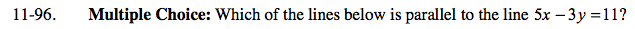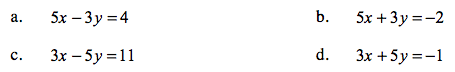Home > AC > Chapter 11 > Lesson 11.2.2 > Problem11-96

11-96.
1. Multiple Choice: Which of the lines below is parallel to the line 5x − 3y = 11? Homework Help ✎

1. 5x − 3y = 4

2. 5x + 3y = − 2

3. 3x − 5y = 11

4. 3x + 5y = − 1Find the equation that has the same slope as the equation given.

Rearrange the equations into slope intercept form and then choose your answer.